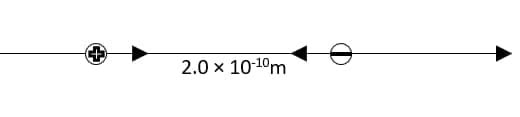If a proton and an electron are released when they are 2.0 \times 10^{- 10}menfurezca3x 2021-11-23 Answered
If a proton and an electron are released when they are $$\displaystyle{2.0}\times{10}^{{-{10}}}{m}$$ apart (a typical atomic distance), find the initial acceleration of each particle.

• Questions are typically answered in as fast as 30 minutes

Solve your problem for the price of one coffee

• Math expert for every subject
• Pay only if we can solve itLouis Gregory

Step 1
Given
We are given the distance between a proton and an electron $$\displaystyle{r}={2.0}\times{10}^{{-{10}}}{m}$$. We are asked to calculate initial acceleration of each particle.
Solution
The two particles have an opposite charge, therefore each one exerts a force F on the other particle. This force could be calculated by the Coulomb's law as in equalion 21.2
$$F=\frac{1}{4\pi\epsilon_{0}}\frac{\mid q_{p}q_{e}\mid}{r^{2}}$$
Where $$\displaystyle\epsilon_{{{0}}}$$ is the electric constant and the term $$\displaystyle{\frac{{{1}}}{{{4}\pi\epsilon_{{{0}}}}}}$$ equals $$\displaystyle{9.0}\times{10}^{{{9}}}{N}\cdot\frac{{m}^{{{2}}}}{{C}^{{{2}}}}$$. $$\displaystyle{q}_{{{p}}}$$ is the charge of proton and equals $$\displaystyle{1.60}\times{10}^{{-{19}}}{C}$$ and $$\displaystyle{q}_{{{e}}}$$, is the charge of electron and equals $$\displaystyle{1.60}\times{10}^{{-{19}}}{C}$$ (See Appendix F)
To obtain the initial acceleration a of the two particles we should get the force that exerted on the proton and the electron. Where the acceleration a is given by Newton's second law in the form.
$$\displaystyle{a}={\frac{{{F}}}{{{m}}}}$$
Where m is the mass of the particle, and for proton $$\displaystyle{m}_{{{p}}}={1.67}\times{10}^{{-{19}}}{k}{g}$$ while for electron $$\displaystyle{m}_{{{e}}}={9.11}\times{10}^{{-{31}}}{k}{g}$$ (See Appendix F)
Now we can plug our values tor $$\displaystyle{g}_{{{p}}}$$, $$\displaystyle{g}_{{{e}}}$$ and $$\displaystyle\tau$$ into equation (1) to get F
$$F=\frac{1}{4\pi\epsilon_{0}}\frac{\mid q_{p}q_{e}\mid}{r^{2}}$$
$$\displaystyle={\left({9.0}\times{10}^{{{9}}}{N}\cdot\frac{{m}^{{{2}}}}{{C}^{{{2}}}}\right)}{\frac{{{\left({1.60}\times{10}^{{-{19}}}{C}\right)}^{{{2}}}}}{{{\left({2.0}\times{10}^{{-{10}}}{m}\right)}^{{{2}}}}}}$$
$$\displaystyle={5.76}\times{10}^{{-{9}}}{N}$$
Step 2
Now, use equation (2) to get the acceleration a for each particle using the value of F.
For proton: We can plug our values for F and $$\displaystyle{m}_{{{p}}}$$ into equation (2) to get the initial acceleration a of the proton
$$\displaystyle{a}_{{{p}}}={\frac{{{F}}}{{{m}_{{{p}}}}}}={\frac{{{5.76}\times{10}^{{-{9}}}{N}}}{{{1.673}\times{10}^{{-{27}}}{k}{g}}}}={3.4}\times{10}^{{{18}}}\frac{{m}}{{s}^{{{2}}}}$$
For electron Again plug our values for F and $$\displaystyle{m}_{{{n}}}$$, into equation (2) to gel the initial acceleration a of the electron
$$\displaystyle{a}_{{{e}}}={\frac{{{F}}}{{{m}_{{{p}}}}}}={\frac{{{5.76}\times{10}^{{-{9}}}{N}}}{{{9.109}\times{10}^{{-{31}}}{k}{g}}}}={6.3}\times{10}^{{{21}}}\frac{{m}}{{s}^{{{2}}}}$$

Not exactly what you’re looking for?Ourst1977Proton
$$\displaystyle{m}_{{{p}}}={1.63}\times{10}^{{-{27}}}{k}{g}$$
$$\displaystyle{q}_{{{p}}}={e}={1.602}\times{10}^{{-{19}}}{C}$$
Electron
$$\displaystyle{m}_{{{p}}}={9.109}\times{10}^{{-{31}}}{k}{g}$$
$$\displaystyle{q}_{{{p}}}=-{e}=-{1.602}\times{10}^{{-{19}}}{C}$$
Since the charges are opposite, the Coulon b fuse is attractive.
$$F=\frac{1}{4\pi\epsilon_{0}}\frac{\mid q_{p}q_{e}\mid}{r^{2}}=\left(8.988\times10^{9}\frac{Nm^{2}}{c^{2}}\right)\frac{\left(1.602\times10^{-19}C\right)^{2}}{\left(2.0\times10^{-10}m\right)^{2}}=5.766709788\times 10^{-9}$$
From Newtois Second Law, $$\displaystyle{F}={m}{a}\Rightarrow{a}={\frac{{{F}}}{{{m}}}}$$
$$\displaystyle\therefore$$ acceleration of Proton:
$$\displaystyle{a}_{{{p}}}={\frac{{{F}}}{{{m}_{{{p}}}}}}={\frac{{{5.766709788}\times{10}^{{-{9}}}{N}}}{{{1.673}\times{10}^{{-{27}}}{k}{g}}}}={3.4469}\times{10}^{{{18}}}{\frac{{{m}}}{{{v}^{{{2}}}}}}$$
$$\displaystyle\therefore{a}_{{{p}}}={3.4}\times{10}^{{{18}}}{\frac{{{m}}}{{{v}^{{{2}}}}}}$$
acceleration of electron:
$$\displaystyle{a}_{{{e}}}={\frac{{{F}}}{{{m}_{{{e}}}}}}={\frac{{{5.766709788}\times{10}^{{-{9}}}{N}}}{{{9.109}\times{10}^{{-{31}}}{k}{g}}}}={6.33078}\times{10}^{{{27}}}{\frac{{{m}}}{{{v}^{{{2}}}}}}$$
$$\displaystyle\therefore{a}_{{{p}}}={6.3}\times{10}^{{{18}}}{\frac{{{m}}}{{{v}^{{{2}}}}}}$$